Courses

# Fluid Mechanics, Strength Of Materials - 1

## 15 Questions MCQ Test GATE Mechanical (ME) 2022 Mock Test Series | Fluid Mechanics, Strength Of Materials - 1

Description
This mock test of Fluid Mechanics, Strength Of Materials - 1 for GATE helps you for every GATE entrance exam. This contains 15 Multiple Choice Questions for GATE Fluid Mechanics, Strength Of Materials - 1 (mcq) to study with solutions a complete question bank. The solved questions answers in this Fluid Mechanics, Strength Of Materials - 1 quiz give you a good mix of easy questions and tough questions. GATE students definitely take this Fluid Mechanics, Strength Of Materials - 1 exercise for a better result in the exam. You can find other Fluid Mechanics, Strength Of Materials - 1 extra questions, long questions & short questions for GATE on EduRev as well by searching above.
QUESTION: 1

Solution:
QUESTION: 2

### A turbine develops 2000 hp under a head of 150 m while running at 300 rpm. What is the specific speed?

Solution: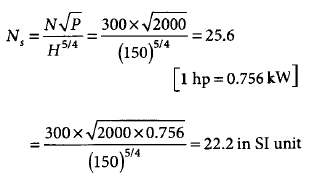QUESTION: 3

### Match List I with List II and select the correct answer using the codes given below the lists: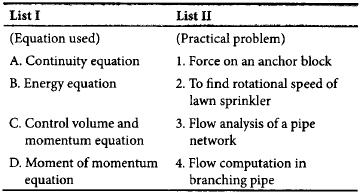Codes: ABCD

Solution:
QUESTION: 4

Match List I with List II and select the correct answer using the codes given below the lists: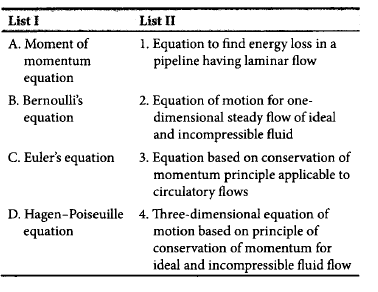Codes:
ABCD

Solution:
QUESTION: 5

When two identical centrifugal pumps are operating in series on a common rising main, then

Solution:
QUESTION: 6

The stream function of a two dimensional flow is given by ψ = 2xy.  the resultant velocity at the point P(2,3) is

Solution: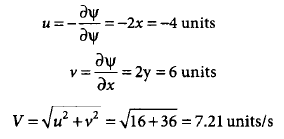QUESTION: 7

Two long pipes in parallel are used to carry water between two reservoirs. The diameter of one pipe is twice that of the other. Both the pipes have the same value of friction factor. Neglect minor losses. What is the ratio of flow rates through the two pipes?

Solution:
QUESTION: 8

The velocity distribution for flow over a plate is given by u = 0.5y - y2, where u is the velocity in m/s at a distance y m above the plate. If the dynamic viscosity of the fluid is 0.9 Ns/m2, then what is the shear stress at 0.20 m from the boundary?

Solution: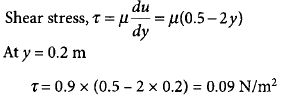QUESTION: 9

The head loss in a pipe of diameter d carrying oil at a flow rate Q over a distance l is h. The pipe is replaced by another with half the diameter, all other things remaining the same. The head loss in this case will be

Solution: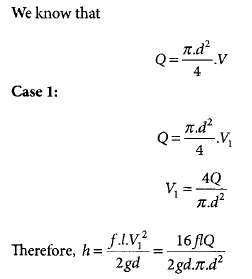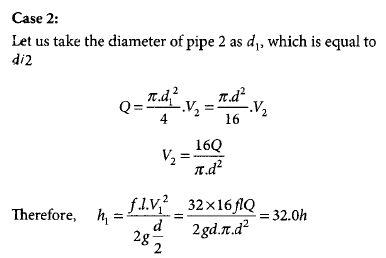*Answer can only contain numeric values
QUESTION: 10

hydraulic turbine has a discharge of 5 m3/s, when operating under a head of 20 m with a speed of 500 rpm. If it is to operate under a head of 15 m, for the same discharge, the rotational speed in rpm will approximately be

Solution:
QUESTION: 11

A cylindrical vessel with a constant plane area of 1 m2 is rotated about its vertical axis such that the liquid inside the vessel is about to spill. If the height of the vessel is 2 m and the height of the paraboloid at 1 m, then the volume (in m3) of the liquid in the vessel will be

Solution:
QUESTION: 12

In steady flow of a compressible fluid through a pipe, the density, area, and velocity at a particular section are 1.5 kg/m3, 0.5 m2, and 3 m/s, respectively. At another section, the density and area are 0.75 kg/m3 and 1.0 m2, respectively. What is the velocity at this section?

Solution: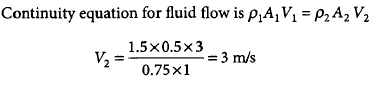QUESTION: 13

The following statements relate to the pressure exerted by a fluid on a submerged curved surface.
1.The vertical component of hydrostatic force acting on a submerged curved surface acts through the center of volume of the fluid directly above the submerged area.
2.The horizontal component of the force acting on a curved surface is the hydrostatic force acting on the vertical projection of the curved surface.
3.The resultant force on a curved surface acts on the bottom of the curved surface.
Of these statements,

Solution:
QUESTION: 14

Match List I with List II and select the correct answer using the codes given below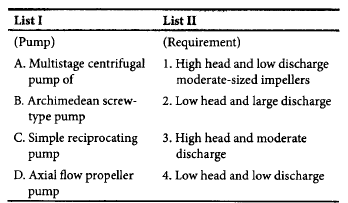Codes  - ABCD

Solution:
QUESTION: 15

Consider the following statements relating to hydraulic gradient line and energy gradient line:
1.In the case of a fluid flowing in a pipeline, hydraulic gradient line and energy gradient line may coincide.
2.The line joining the points representing piezometric heads is known as hydraulic gradient.
In the case of ideal fluid, energy gradient line is always horizontal.
3.Hydraulic gradient line has a downward slope in the case of flow through pipes.
Of these statements,

Solution: